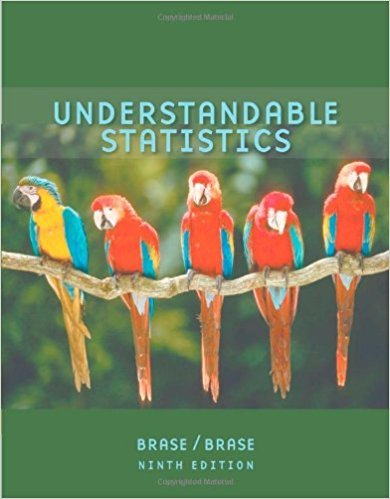×
×

# Solutions for Chapter 10.1: CORRELATION AND REGRESSION## Full solutions for Understandable Statistics | 9th Edition

ISBN: 9780618949922Solutions for Chapter 10.1: CORRELATION AND REGRESSION

Solutions for Chapter 10.1
4 5 0 374 Reviews
27
1
##### ISBN: 9780618949922

Understandable Statistics was written by and is associated to the ISBN: 9780618949922. Chapter 10.1: CORRELATION AND REGRESSION includes 22 full step-by-step solutions. This expansive textbook survival guide covers the following chapters and their solutions. Since 22 problems in chapter 10.1: CORRELATION AND REGRESSION have been answered, more than 33574 students have viewed full step-by-step solutions from this chapter. This textbook survival guide was created for the textbook: Understandable Statistics, edition: 9.

Key Statistics Terms and definitions covered in this textbook
• Acceptance region

In hypothesis testing, a region in the sample space of the test statistic such that if the test statistic falls within it, the null hypothesis cannot be rejected. This terminology is used because rejection of H0 is always a strong conclusion and acceptance of H0 is generally a weak conclusion

• All possible (subsets) regressions

A method of variable selection in regression that examines all possible subsets of the candidate regressor variables. Eficient computer algorithms have been developed for implementing all possible regressions

• Average

See Arithmetic mean.

• Categorical data

Data consisting of counts or observations that can be classiied into categories. The categories may be descriptive.

• Chi-square test

Any test of signiicance based on the chi-square distribution. The most common chi-square tests are (1) testing hypotheses about the variance or standard deviation of a normal distribution and (2) testing goodness of it of a theoretical distribution to sample data

• Coeficient of determination

See R 2 .

• Components of variance

The individual components of the total variance that are attributable to speciic sources. This usually refers to the individual variance components arising from a random or mixed model analysis of variance.

• Conditional variance.

The variance of the conditional probability distribution of a random variable.

• Consistent estimator

An estimator that converges in probability to the true value of the estimated parameter as the sample size increases.

• Continuous distribution

A probability distribution for a continuous random variable.

• Continuous random variable.

A random variable with an interval (either inite or ininite) of real numbers for its range.

• Correlation coeficient

A dimensionless measure of the linear association between two variables, usually lying in the interval from ?1 to +1, with zero indicating the absence of correlation (but not necessarily the independence of the two variables).

• Correlation matrix

A square matrix that contains the correlations among a set of random variables, say, XX X 1 2 k , ,…, . The main diagonal elements of the matrix are unity and the off-diagonal elements rij are the correlations between Xi and Xj .

• Critical value(s)

The value of a statistic corresponding to a stated signiicance level as determined from the sampling distribution. For example, if PZ z PZ ( )( .) . ? =? = 0 025 . 1 96 0 025, then z0 025 . = 1 9. 6 is the critical value of z at the 0.025 level of signiicance. Crossed factors. Another name for factors that are arranged in a factorial experiment.

• Deming

W. Edwards Deming (1900–1993) was a leader in the use of statistical quality control.

• Empirical model

A model to relate a response to one or more regressors or factors that is developed from data obtained from the system.

• Event

A subset of a sample space.

• Exponential random variable

A series of tests in which changes are made to the system under study

• Gaussian distribution

Another name for the normal distribution, based on the strong connection of Karl F. Gauss to the normal distribution; often used in physics and electrical engineering applications

• Generating function

A function that is used to determine properties of the probability distribution of a random variable. See Moment-generating function

×